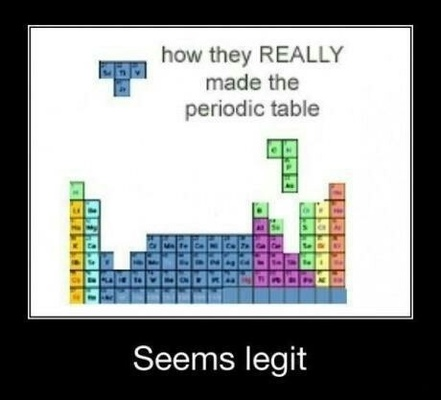Electron Configurations
4 years ago
cvoigt
Save
Edit
Host a game
Live GameLive
Homework
Solo Practice
Practice27 QuestionsShow answers
• Question 1
30 seconds
Q. What atom matches this electron configuration?
1s22s22p63s2
Neon
Magnesium
Aluminum
Potassium
• Question 2
30 seconds
Q. What atom matches this electron configuration?
1s22s22p63s23p64s23d10
Zinc
Copper
Nickel
Germanium
• Question 3
30 seconds
Q. What is the electron configuration for a Sulfur atom?
1s22s22p63p6
1s22s22p63s23p6
1s22s22p63s23p4
3p4
• Question 4
30 seconds
Q. What atom matches this electron configuration?
1s22s22p63s33p64s23d104p65s24d105p66s24f145d9
Mercury
Gold
Platinum
Thallium
• Question 5
30 seconds
Q. What electron configuration matches an oxygen atom?
1s22s22p63s2, 3p64s23d104p5
1s22s22p4
1s22s22p6
1s22s22p63s23p64s23d1
• Question 6
30 seconds
Q. Which of the following is the smallest in size?
N
S
I
Fr
• Question 7
30 seconds
Q. Which of the following is the smallest in size?
K
Na
Li
Cs
• Question 8
60 secondsQ. How many electrons does Si contain? (click to see image)
14
28
2
4
• Question 9
60 secondsQ. How many valence electrons does Si contain? (click to see image)
14
28
2
4
• Question 10
60 seconds
Q. How many electrons can the first energy level hold?
1
2
8
0
• Question 11
60 seconds
Q. An orbital can at most hold how many electrons?
1 electron
2 electrons
3 electrons
4 electrons
• Question 12
60 seconds
Q. The electron configuration of an atom is 1s22s22p6.  The number of electrons in the atom is
3
6
8
10
• Question 13
60 seconds
Q. The electron configuration of an atom is 1s22s22p6.  The number of valence electrons in the atom is
3
6
8
10
• Question 14
30 secondsQ. What atom is represented here?
Carbon
Nitrogen
Oxygen
Fluorine
• Question 15
30 secondsQ. How many valence electrons are represented here?
7
5
2
8
• Question 16
30 secondsQ. What is the electron configuration for this atom?
1s22s22p6
1s22s22p5
1s22s22p3
2s22p3
• Question 17
30 secondsQ. What is the highest occupied energy level?
1
2
3
4
• Question 18
30 secondsQ. What is the highest occupied energy level?
4
5
6
7
• Question 19
30 secondsQ. How many valence electrons are there?
1
2
8
79
• Question 20
30 seconds
Q. How many orbitals are in the 4s sublevel?
1
2
3
4
• Question 21
30 seconds
Q. How many orbitals are in the 4p sublevel?
2
3
4
6
• Question 22
30 seconds
Q. How many orbitals are in the 4d sublevel?
2
4
5
10
• Question 23
30 seconds
Q. How many orbitals are in the 4f sublevel?
2
4
7
14
• Question 24
30 seconds
Q. After filling 5s, electrons would fill...
5p
4d
5d
3f
• Question 25
30 seconds
Q. After filling 6s, electrons would fill
4f
5d
6p
7g
• Question 26
30 secondsQ. How many unshared pairs of electrons are in this orbital diagram? (click to see image?
2
3
5
15
• Question 27
30 secondsQ. How many unshared pairs of electrons are in this orbital diagram? (click to see image)